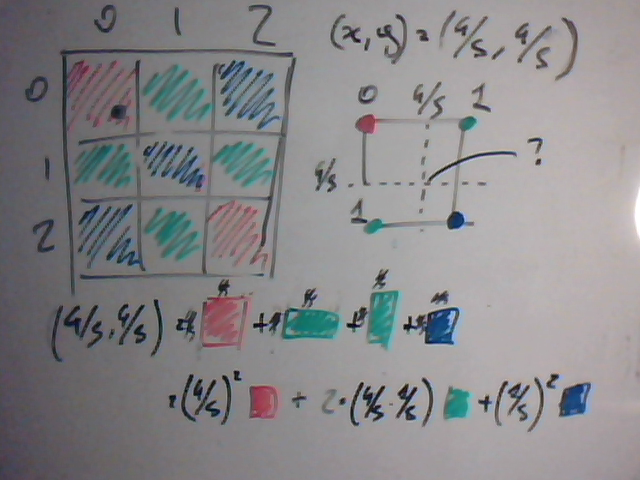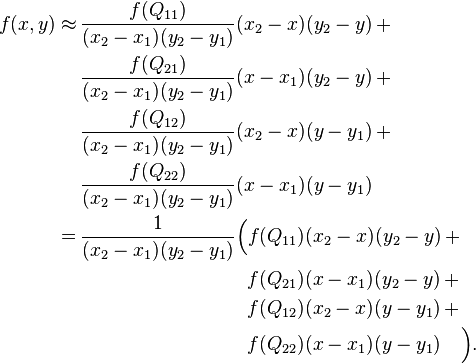Here we are again.
We left talking about transforms and rotations.
See, all in there is good and well, but there is a slight problem in practice I hinted at.

If you remember we said how the fact that we are working in the discrete poses a problem: we have to approximate real coordintes of the destination point with integer coordinates – this, using the naive method, nearest neighbor approximation (aka: simply rounding to the next pixel) causes aliasing.

That’s where interpolation kicks in.

A warning for those unaware: once again, signal processing is terribly big field and everything has vast theoretical implications (and vast practical applications too, e.g. most concepts apply to audio processing as well).
We deal with the matter in an extremely practical fashion, hence the post title.

Now – specifically, we deal with bilinear transform.

Intuitively, the idea is: we try to infer what colour would be a pixel at the given coordinates if there actually were one.
We can’t add new information – this is immediately apparent by the fact that interpolated transforms are inevitably slightly “blurry” -, but we can eliminate the aliasing caused by abruptly rounding to the closest value.

If I am not mistaken, “abruptly” has a special meaning in this context, and means: “upper bound in frequency as per the Shannon-Nyquist theorem”: http://en.wikipedia.org/wiki/Nyquist%E2%80%93Shannon_sampling_theorem

Now, let’s get going. Look at this:(You will excuse me for posting a pic of a whiteboard, but my parallel scanner from 1997 has apprently just died)

In the example, out projected source is (4/5, 4/5).
What would look like a pixel in (4/5, 4/5)?
We don’t really know, but we can estimate its color to be a weighted average of its neighbors.

So, the math (from Wikipedia):And now let’s see some code from transparent_surface.cpp – this time you don’t get the pseudocode since it’s really a matter of carrying out the above and checking for a few edge cases and avoiding division by zero:

```void TransparentSurface::copyPixelBilinear(float projX, float projY, int dstX, int dstY, const Common::Rect &srcRect, const Common::Rect &dstRect, const TransparentSurface *src, TransparentSurface *dst) {
int srcW = srcRect.width();
int srcH = srcRect.height();
int dstW = dstRect.width();
int dstH = dstRect.height();

assert(dstX >= 0 && dstX < dstW);
assert(dstY >= 0 && dstY < dstH);

float x1 = floor(projX);
float x2 = ceil(projX);
float y1 = floor(projY);
float y2 = ceil(projY);

uint32 Q11, Q12, Q21, Q22;

if (x1 >= srcW || x1 < 0 || y1 >= srcH || y1 < 0) {
Q11 = 0;
} else {
Q11 = READ_UINT32((const byte *)src->getBasePtr((int)(x1 + srcRect.left),(int)(y1 + srcRect.top)));
}

if (x1 >= srcW || x1 < 0 || y2 >= srcH || y2 < 0) {
Q12 = 0;
} else {
Q12 = READ_UINT32((const byte *)src->getBasePtr((int)(x1 + srcRect.left), (int)(y2 + srcRect.top)));
}

if (x2 >= srcW || x2 < 0 || y1 >= srcH || y1 < 0) {
Q21 = 0;
} else {
Q21 = READ_UINT32((const byte *)src->getBasePtr((int)(x2 + srcRect.left), (int)(y1 + srcRect.top)));
}

if (x2 >= srcW || x2 < 0 || y2 >= srcH || y2 < 0) {
Q22 = 0;
} else {
Q22 = READ_UINT32((const byte *)src->getBasePtr((int)(x2 + srcRect.left), (int)(y2 + srcRect.top)));
}

byte *Q11s = (byte *)&Q11;
byte *Q12s = (byte *)&Q12;
byte *Q21s = (byte *)&Q21;
byte *Q22s = (byte *)&Q22;

uint32 color;
byte *dest = (byte *)&color;

float q11x = (x2 - projX);
float q11y = (y2 - projY);
float q21x = (projX - x1);
float q21y = (y2 - projY);
float q12x = (x2 - projX);
float q12y = (projY - y1);

if (x1 == x2 && y1 == y2) {
for (int c = 0; c < 4; c++) {
dest[c] = ((float)Q11s[c]);
}
} else {

if (x1 == x2) {
q11x = 0.5;
q12x = 0.5;
q21x = 0.5;
} else if (y1 == y2) {
q11y = 0.5;
q12y = 0.5;
q21y = 0.5;
}

for (int c = 0; c < 4; c++) {
dest[c] = (byte)(
((float)Q11s[c]) * q11x * q11y +
((float)Q21s[c]) * q21x * q21y +
((float)Q12s[c]) * q12x * q12y +
((float)Q22s[c]) * (1.0 -
q11x * q11y -
q21x * q21y -
q12x * q12y)
);
}
}
WRITE_UINT32((byte *)dst->getBasePtr(dstX + dstRect.left, dstY + dstRect.top), color);
}
```